NEET  >  NCERT Based Test: Motion in a Magnetic Field

# NCERT Based Test: Motion in a Magnetic Field

Test Description

## 5 Questions MCQ Test Physics Class 12 | NCERT Based Test: Motion in a Magnetic Field

NCERT Based Test: Motion in a Magnetic Field for NEET 2023 is part of Physics Class 12 preparation. The NCERT Based Test: Motion in a Magnetic Field questions and answers have been prepared according to the NEET exam syllabus.The NCERT Based Test: Motion in a Magnetic Field MCQs are made for NEET 2023 Exam. Find important definitions, questions, notes, meanings, examples, exercises, MCQs and online tests for NCERT Based Test: Motion in a Magnetic Field below.
Solutions of NCERT Based Test: Motion in a Magnetic Field questions in English are available as part of our Physics Class 12 for NEET & NCERT Based Test: Motion in a Magnetic Field solutions in Hindi for Physics Class 12 course. Download more important topics, notes, lectures and mock test series for NEET Exam by signing up for free. Attempt NCERT Based Test: Motion in a Magnetic Field | 5 questions in 5 minutes | Mock test for NEET preparation | Free important questions MCQ to study Physics Class 12 for NEET Exam | Download free PDF with solutions
 1 Crore+ students have signed up on EduRev. Have you?
NCERT Based Test: Motion in a Magnetic Field - Question 1

### A charged particle is moving on circular path with velocity v in a uniform magnetic field B, if the velocity of the charged particle is doubled and strength of Magnetic field is halved, then radius becomes

Detailed Solution for NCERT Based Test: Motion in a Magnetic Field - Question 1

As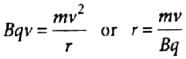According to the question, v‘ = 2v and B' = B/2
∴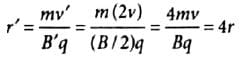NCERT Based Test: Motion in a Magnetic Field - Question 2

### An electron of energy 1800 eV describes a circular path in magnetic field of flux density 0.4 T. The radius of path is (q = 1.6 x 10-19 C, me = 9.1 x 10-31 kg)

Detailed Solution for NCERT Based Test: Motion in a Magnetic Field - Question 2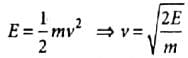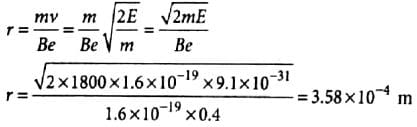NCERT Based Test: Motion in a Magnetic Field - Question 3

### Two α-partides have the ratio of their velocities as 3 : 2 on entering the field. If they move in different circular paths, then the ratio of the radii of their paths is

Detailed Solution for NCERT Based Test: Motion in a Magnetic Field - Question 3

As qvB = mv2/r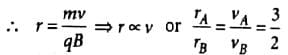NCERT Based Test: Motion in a Magnetic Field - Question 4

When a positively charged particle enters a uniform magnetic field with uniform velocity, its trajectory can be (i) a straight line (ii) a circle (iii) a helix.

NCERT Based Test: Motion in a Magnetic Field - Question 5

Two particles of equal charges after being accelerated through the same potential difference enter in a uniform transverse magnetic field and describe circular paths of radii Rand R2. Then the ratio of their respective masses (M1/M2) is

Detailed Solution for NCERT Based Test: Motion in a Magnetic Field - Question 5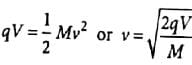And Bqv = Mv2/R Or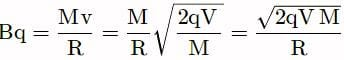Using (i)
or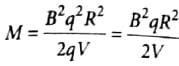∴ M ∝ R2 (∵ B, q  and V are same for the given two particles)
Hence (M1/M2) = (R1/R2)2

## Physics Class 12

157 videos|452 docs|213 tests
 Use Code STAYHOME200 and get INR 200 additional OFF Use Coupon Code
Information about NCERT Based Test: Motion in a Magnetic Field Page
In this test you can find the Exam questions for NCERT Based Test: Motion in a Magnetic Field solved & explained in the simplest way possible. Besides giving Questions and answers for NCERT Based Test: Motion in a Magnetic Field, EduRev gives you an ample number of Online tests for practice

## Physics Class 12

157 videos|452 docs|213 tests

### How to Prepare for NEET

Read our guide to prepare for NEET which is created by Toppers & the best Teachers